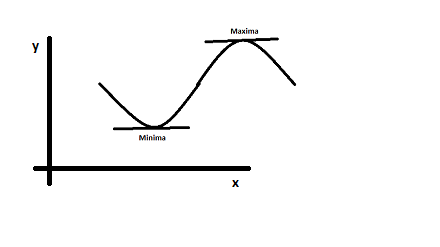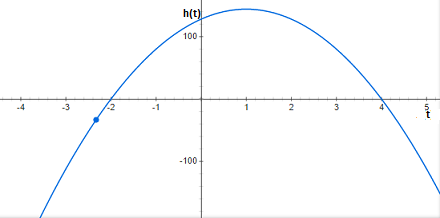# An object is thrown straight up into the air at an initial vertical velocity of 32 feet per...

## Question:

An object is thrown straight up into the air at an initial vertical velocity of 32 feet per second from an initial height of 128 feet and is represented by the vertical motion model, {eq}h(t) = -16t^2 + 32t + 128 {/eq}. How much time does it take for the object to reach its maximum height?

## Finding maxima and minima using differentiation

Differentiation is a tool that can be used to find out the maxima or minima of an equation.As you can see from the figure, the tangent vectors at maxima or minima are parallel to the x-axis i.e., their slope is zero.

This implies that the derivate of a function is zero at maxima or minima.

For maxima, the double derivative will be negative, and for minima, the double derivative will be positive.

{eq}h(t)=-16t^{2}+32t+128 {/eq}

{eq}\frac{\mathrm{d} h(t)}{\mathrm{d} t} = \frac{\mathrm{d} (-16t^{2}+32t+128)}{\mathrm{d} t} {/eq}

{eq}\frac{\mathrm{d} h(t)}{\mathrm{d} t}= \frac{\mathrm{d} (-16t^{2})}{\mathrm{d} t}+\frac{\mathrm{d} (32t)}{\mathrm{d} t}+\frac{\mathrm{d} (128)}{\mathrm{d} t} {/eq}

{eq}{h}'(t)= -32t+32\ \ ...... {/eq}1

For maxima or minima, {eq}\frac{\mathrm{d} {h}'(t)}{\mathrm{d} t} = 0 {/eq}

{eq}\Rightarrow \frac{\mathrm{d} {h}'(t)}{\mathrm{d} t}= -32t+32=0 {/eq}

{eq}\Rightarrow t=1 {/eq}

To confirm that we have maxima at {eq}\displaystyle{ t=1 }{/eq}, we double differentiate h(t).

{eq}\frac{\mathrm{d} {h}'(t)}{\mathrm{d} t}= \frac{\mathrm{d} (-32t+32)}{\mathrm{d} t} {/eq}

{eq}\frac{\mathrm{d} {h}'(t)}{\mathrm{d} t} = \frac{\mathrm{d} (-32t)}{\mathrm{d} t}+\frac{\mathrm{d} (32)}{\mathrm{d} t} {/eq}

{eq}{h}''(t)= -32 {/eq}

At {eq}\displaystyle{ t=1 }{/eq},

{eq}{h}''(1)<0 {/eq}

This implies that we have maxima at {eq}\displaystyle{ t=1 }{/eq}. In this question, the maxima obtained is also the maximum as can be seen from the graph of this equation.Therefore, It takes 1 second for the object to reach its maximum height.